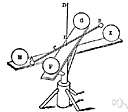# gravitational constant

(redirected from Big Gee)
Also found in: Thesaurus, Medical, Encyclopedia.

## gravitational constant

n. Abbr. G
The constant relating the force of gravitational attraction between two bodies to the product of their masses and the inverse square of the distance between them in Newton's law of gravitation. It equals 6.67 × 10-11 m3kg-1s-2.

## gravitational constant

n
(General Physics) the factor relating force to mass and distance in Newton's law of gravitation. It is a universal constant with the value 6.673 × 10–11 N m2 kg–2. Symbol: G

## grav·i·ta·tion·al constant

(grăv′ĭ-tā′shə-nəl)
A number used to calculate the force of the gravitational attraction between two bodies in Newton's law of gravitation. The gravitational constant equals 6.67 × 10-11 cubic meters per kilogram per second squared. See more at Newton's law of gravitation.
ThesaurusAntonymsRelated WordsSynonymsLegend:
 Noun 1gravitational constant - (physics) the universal constant relating force to mass and distance in Newton's law of gravitationlaw of gravitation, Newton's law of gravitation - (physics) the law that states any two bodies attract each other with a force that is directly proportional to the product of their masses and inversely proportional to the square of the distance between themnatural philosophy, physics - the science of matter and energy and their interactions; "his favorite subject was physics"constant - a number representing a quantity assumed to have a fixed value in a specified mathematical context; "the velocity of light is a constant"
Translations
Gravitationskonstante
References in periodicals archive ?
It is clear that the sport which an awful lot of people still really love does need a big gee up.
Just three points behind the pair in the race for the pounds 15,000 first prize is Big Gee, from Essex, who would overtake the front two if Kasbah Bliss, one of his bankers, obliges in today 's Ladbrokes World Hurdle.
never mind england and the world cup this is the big one boro and the Uefa cup come on boro big gee norton

Site: Follow: Share:
Open / Close